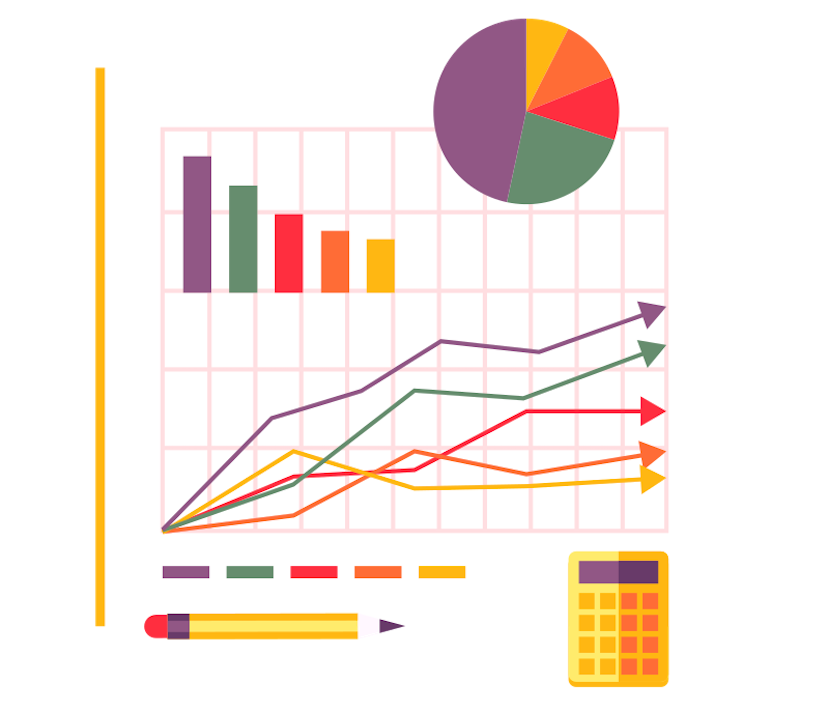# Practicing Probability

#### Do you teach probability theory? Help your students understand probability by offering them online exercises to practice with. Starting with the basics up to conditional probability.## Open Exercises

Below you find a list of all the lessons and exercises about probability (theory), that are currently already available in Grasple. These materials have been created by Grasple over the past five years in collaboration with different universities. All of these will be released with an open Creative Commons license in the coming months. To access materials that are not yet CC licensed, you can create a free teacher account.

#### Probability

• 9

Probability Trees

• 4

Conditional Probability

• 4

Take With or Without Replacement

• 5

Expected Value

• 6

Rules for Repetition

#### Probability Basics

• 6

Conceptual Understanding of Random Variables

• 7

Conceptual Understanding of Probabilities

• 7

Conceptual Understanding of Sample Space

• 7

Conceptual Understanding of (Probability) Distributions

• 7

Conceptual Understanding of Discrete vs Continuous Distributions

• 7

Conceptual Understanding of Probability Density Functions and Cumulative Density Functions

#### Probability Calculations & Rules

• 7

Conceptual Understanding of Marginal Probabilities

• 7

Conceptual Understanding of Joint Probabilities

• 7

Conceptual Understanding of Conditional Probabilities

• 7

Notation of Probabilities

• 7

Conceptual Understanding of Dependent and Independent Events

• 7

Conceptual Understanding of Probability Rules

• 4

Computing Probabilities Using Probability Rules

#### Counting and Expected Values

• 7

Conceptual Understanding of Expected Values

• 6

Computing Expected Values

• 7

Conceptual Understanding of Combinations

• 7

Conceptual understanding of Permutations

• 6

Computing Combinations

• 3

Computing Permutations

#### The Normal Distribution

• 5

Notation of Normal Distribution and Parameters

• 7

Conceptual Understanding of Standard Normal Distribution

• 3

Conceptual Understanding of Combining of Several Normally Distributed Variables

• 5

Computing Parameters of Combined Normally Distributed Variables

#### Binomial Distribution

• 12

Conceptual Understanding of Binomial Distribution

• 5

Notation of Binomial Distribution and Parameters

• 8

Properties of Binomial Distributions

• 3

Computing with Binomial Distributions

#### Poisson Distribution

• 7

Conceptual Understanding of Poisson Distribution

• 7

Notation of Poisson Distribution and Parameters

• 5

Properties of the Poisson Distribution

• 4

Computing with Poisson Distributions

• 12

Central Limit Theorem

#### t-distribution

• 10

Student's t-Distribution (conceptual)

• 5

t-Distribution

#### Random Variables

• 2

Population and Sample - Conceptual explanation

• 3

Experiments - Conceptual Explanation

• 2

Random Variables - Conceptual Explanation

• 3

Realizations of Random Variables - Conceptual Explanation

• 3

Continuous versus Discrete Random Variables - Conceptual Explanation

• 2

Notation of Probabilities - Conceptual Explanation

#### Distribution Functions

• 2

Probability Distribution - Conceptual Explanation

• 2

Distribution Functions - Conceptual Explanation

• 3

Probability Density Functions - Conceptual Explanation

• 2

Probability Calculations Using Probability Density Functions

• 2

Probability Mass Functions - Conceptual Explanation

• 2

Probability Calculations Using Probability Mass Functions

#### Distribution Functions

• 3

Cumulative Distribution Functions - Conceptual Explanation

• 2

Probability Calculations Using Cumulative Distribution Functions

• 2

Relation and Difference Between Mass, Density and Cumulative Functions - Conceptual Explanation

• 2

Finding Probability Density Function Using the Cumulative Distribution Function

#### Well known distribution functions

• 1

Well Known Continuous Distribution Functions (List)

#### Center and Spread of Distributions

• 4

Expected Value - Conceptual Explanation

• 4

Calculating the Expected Value for Continuous Random Variables

• 4

Second Moment - Conceptual Explanation

• 4

Calculating the Second Moment for Continuous Random Variables

• 4

Variance - Conceptual Explanation

• 4

Calculating the Variance of a Random Variable

#### Conditional Probability

• 2

Conditional Probability - Conceptual Explanation

• 2

Conditional Distribution Functions - Conceptual Explanation

• 5

Calculations with Conditional Distribution Functions

#### Estimation

• 2

Statistic versus Parameter - Conceptual Explanation

• 3

Statistical Models and Estimation - Conceptual Explanation

• 3

Regression Analysis - Conceptual Explanation

• 2

Finding Parameters of Linear Model Using Regression Analysis

• 2

Maximum Likelihood - Conceptual Explanation

• 5

Estimate Parameters of Distributions Using Maximum Likelihood Estimation

##### Legend

Indicates whether a lesson/explanation is available per subject
10 Indicates if and how many exercises are currently available per subject
Content has an open Creative Commons license
Content will be released with an open Creative Commons license in the near future

## Looking for other subjects?

As you can see, we have an extensive list of probability exercises. But there’s more! Click on the topics below to view more openly licensed practice material, or simply create your own.

We make Math and Statistics Education more engaging by offering an online practice platform. With this, educators can create interactive exercises, and students can practice these exercises while receiving immediate feedback on their efforts.